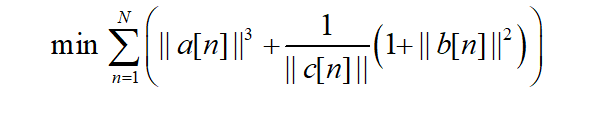# Cannot perform the operation: {convex} .* {convex}

Hi,
My objective function isand the constraints are just linear inequality constraints. It’ a convex problem.
I am having quite some trouble to implement this function. The error I get is “Cannot perform the operation {convex}{convex}”. I wrote it as below,
for n=1:N
E(1,n)=pow_pos(norm(a(:,n)),3) + inv_pos(c(1,n))
(1+pow_pos(norm(b(:,n)),2));
end
minimize sum(E)

Any suggestions on how to improve the code are welcome as well.

Thanks

1 Like

Use `quad_over_lin` for ||b(n)||^2/max(c,0) if that indeed matches what you want.

1 Like

where b is a matrix of 2 rows and N columns and c is a vector of 1 row and N columns
But there is still an error：The first argument must be affine.

`quad_over_lin(b(:,n),c(n))`

quad_over_lin Sum of squares over linear.
Z=quad_over_lin(X,Y), where X is a vector and Y is a scalar, is equal to
SUM(ABS(X).^2)./Y if Y is positive, and +Inf otherwise. Y must be real.

``````If X is a matrix, quad_over_lin(X,Y) is a row vector containing the values
of quad_over_lin applied to each column. If X is an N-D array, the operation
is applied to the first non-singleton dimension of X.

quad_over_lin(X,Y,DIM) takes the sum along the dimension DIM of X.
A special value of DIM == 0 is accepted here, which is automatically
replaced with DIM == NDIMS(X) + 1. This has the effect of eliminating
the sum; thus quad_over_lin( X, Y, NDIMS(X) + 1 ) = ABS( X ).^2 ./ Y.

In all cases, Y must be compatible in the same sense as ./ with the squared
sum; that is, Y must be a scalar or the same size as SUM(ABS(X).^2,DIM).

Disciplined convex programming information:
quad_over_lin is convex, nonmontonic in X, and nonincreasing in Y.
Thus when used with CVX expressions, X must be convex (or affine)
and Y must be concave (or affine).``````